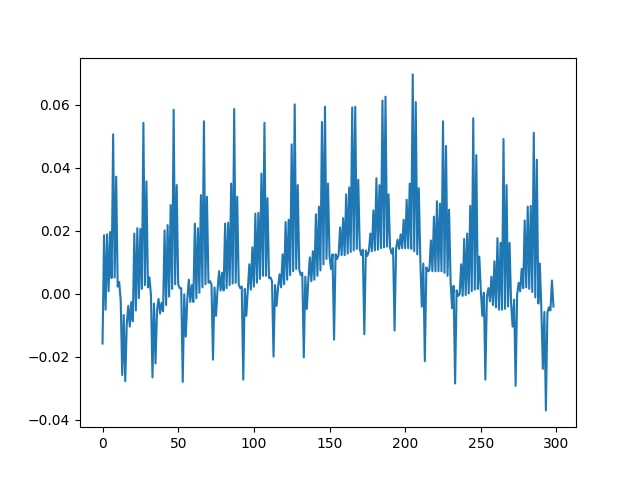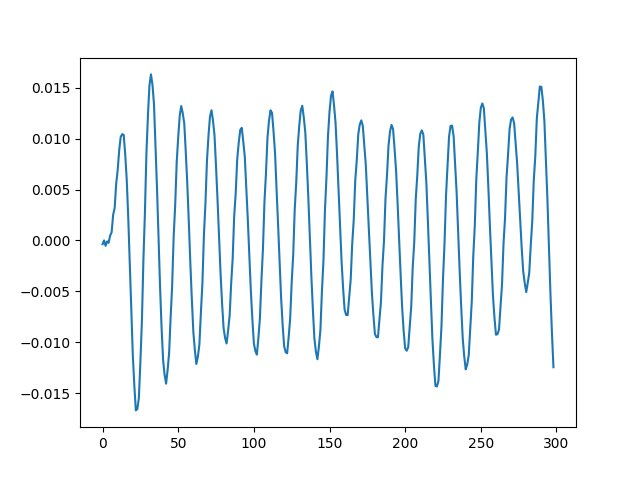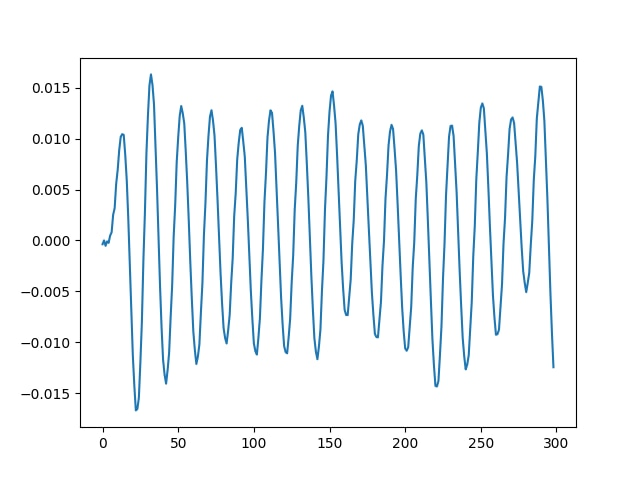## How to implement biquads to filter an Electrocardiography signal

In this guide, we will see how to filter an Electrocardiography (ECG) signal using a biquad filter defined with SciPy and by calling the CMSIS-DSP functions from Python. We will check that we get the same results with both methods. The biquad filter will remove the high frequency noise. This guide won't explain how to design such a biquad filter.

You can see a a sample ECG signal in the following image (generated with Python):We want to remove the high frequency noise from the signal.

First, let’s import some libraries that we will need for our example:

```import cmsisdsp as dsp
import numpy as np
from scipy import signal
from pylab import figure, plot, show```

Here is an implementation of the filter in SciPy, which shows three biquad filters in sequence. The biquads are described with their poles and zeros.

```p0 = np.exp(1j*0.05) * 0.98
p1 = np.exp(1j*0.25) * 0.9
p2 = np.exp(1j*0.45) * 0.97

z0 = np.exp(1j*0.02)
z1 = np.exp(1j*0.65)
z2 = np.exp(1j*1.0)
g = 0.02```

With those poles and zeros, the filter can be created with:

```sos = signal.zpk2sos(
[z0,np.conj(z0),z1,np.conj(z1),z2,np.conj(z2)]
,[p0, np.conj(p0),p1, np.conj(p1),p2, np.conj(p2)]
,g)```

Now, we filter the signal with the biquad filter and display the first 300 samples of the result (nb is equal to 300).

```res=signal.sosfilt(sos,sig)
figure()
plot(res[1:nb])```

The high frequency noise has now been removed, as you can see in the following image:Let’s replace this filter with a Q31 implementation using CMSIS-DSP and check that we get the same result at the end.

First, we need to create an instance object. In CMSIS-DSP, an instance object corresponds to a C struct which must be allocated, and which contains the filter coefficients and the filter state. The name of the Python function is the name of the C type in CMSIS-DSP.

` biquadQ31 = dsp.arm_biquad_casd_df1_inst_q31()`

To initialize this instance with the CMSIS-DSP initialization function, we need a state array.

The state array must be big enough. According to the CMSIS-DSP documentation, we need four samples for each biquad. Our example includes three biquad

```numStages=3
state=np.zeros(numStages*4)```

To initialize the instance object, we also need a coefficient array that contains the filter coefficients.

SciPy and CMSIS-DSP do not save the coefficients in the same way in memory. In CMSIS-DSP, the a0 coefficient is assumed to be 1 and is not saved in memory. Also, in CMSIS-DSP the "a" coefficients are negative compared to the SciPy conventions.

So, to use the coefficients with CMSIS-DSP we need to convert them.

Finally, to avoid saturations in the Q31 implementation we need to scale down the coefficients by 4. The following Python lines are doing the conversions:

```coefs=np.reshape(np.hstack((sos[:,:3],-sos[:,4:])),15)
coefs = coefs / 4.0```

And of course, the coefficients need to be converted to Q31 format. The toQ31 is a simple Python function that we wrote to convert the float into an integer using Q31 format.

`coefsQ31 = toQ31(coefs)`

Now that we have our coefficients, we can initialize our filter instance. Since the coefficients were scaled down by 4, we need to set the postshift to 2 in the filter as explained in the CMSIS-DSP documentation.

The API arm_biquad_cascade_df1_init_q31 is a direct copy of the C version and would be used in the same way in the C implementation.

```postshift = 2

Now that the filter instance has been initialized, we are nearly ready to filter our signal. But before testing the filter, we also need to convert the input signal to Q31 format:

`sigQ31=toQ31(sig)`

Now we can filter our Q31 signal using the CMSIS-DSP biquad function. Here we limit the signal to only the first 300 samples (nb is equal to 300).

The API arm_biquad_cascade_df1_q31 is a near copy of the C one. The only difference is that the blockSize parameter is inferred from the signal array to make the API a bit easier to use from Python. Also, the CMSIS-DSP pDst parameter is transformed into a return value, so that the API is easier to use. Apart from these diffferences, the API is a copy of the C API.

`res2=dsp.arm_biquad_cascade_df1_q31(biquadQ31,sigQ31[1:nb])`

The result res2 from the function must be converted back to float before being displayed. This is because res2 is a Q31 signal. Once done, the float version can be displayed to check that the filter is behaving as intended with no saturation issues.

```figure()
plot(res2[1:nb])
show()```

And we see on the picture that we get the intended result:Without this Python API, testing this example would have required much more work, including:

• A C implementation
• Generation of test patterns
• A test framework to load the input test pattern and read the output from the execution environment. It could be a board or simulator.